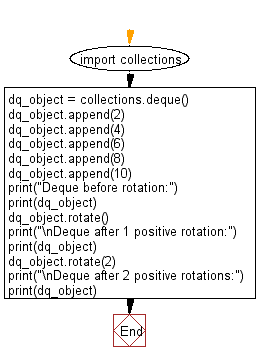﻿ Python: Rotate a Deque Object specified number (positive) of times - w3resource# Python: Rotate a Deque Object specified number (positive) of times

## Python Collections: Exercise-13 with Solution

Write a Python program to rotate a Deque Object specified number (positive) of times.

Sample Solution:

Python Code:

``````import collections
# declare an empty deque object
dq_object = collections.deque()
# Add elements to the deque - left to right
dq_object.append(2)
dq_object.append(4)
dq_object.append(6)
dq_object.append(8)
dq_object.append(10)
print("Deque before rotation:")
print(dq_object)
# Rotate once in positive direction
dq_object.rotate()
print("\nDeque after 1 positive rotation:")
print(dq_object)
# Rotate twice in positive direction
dq_object.rotate(2)
print("\nDeque after 2 positive rotations:")
print(dq_object)
```
```

Sample Output:

```Deque before rotation:
deque([2, 4, 6, 8, 10])

Deque after 1 positive rotation:
deque([10, 2, 4, 6, 8])

Deque after 2 positive rotations:
deque([6, 8, 10, 2, 4])
```

Flowchart:## Visualize Python code execution:

The following tool visualize what the computer is doing step-by-step as it executes the said program:

Python Code Editor:

Have another way to solve this solution? Contribute your code (and comments) through Disqus.

What is the difficulty level of this exercise?

Test your Programming skills with w3resource's quiz.

﻿

## Python: Tips of the Day

The Zip() Function:

```>>> students = ('John', 'Mary', 'Mike')
>>> ages = (15, 17, 16)
>>> scores = (90, 88, 82, 17, 14)
>>> for student, age, score in zip(students, ages, scores):
...     print(f'{student}, age: {age}, score: {score}')
...
John, age: 15, score: 90
Mary, age: 17, score: 88
Mike, age: 16, score: 82
>>> zipped = zip(students, ages, scores)
>>> a, b, c = zip(*zipped)
>>> print(b)
(15, 17, 16)
```